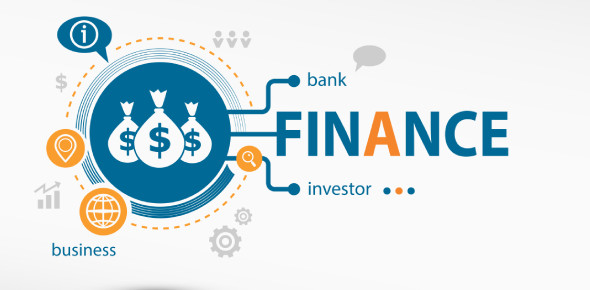# A Test On Finance Concepts! Trivia Quiz

54 Questions | Total Attempts: 582SettingsTest on finance concepts. The cash flow ratio helps to assess how current liabilities are covered by cash inflow in the business. Do you know the formula you can use to assess the quick ratio, cash flow ratio or even net income of a business? The quiz below will be perfect to test it out. Do give it a shot and get to see how well you will do. Good luck!

• 1.
In a comparative analysis of the financial statement, the technique used is
• A.

Graphical analysis

• B.

Preference analysis

• C.

Common size analysis

• D.

Returning analysis

• 2.
The net income available to stockholders Rs 1,250 and total assets Rs.10,960 then return on common equity is
• A.

0.11%

• B.

11.40%

• C.

0.12 times

• D.

12%

• 3.
The price per share Rs.25 and the cash flow per share Rs. 6 then the price to cash flow ratio is
• A.

0.24 times

• B.

4.16 times

• C.

4.16%

• D.

24%

• 4.
The low price to earnings ratio is the result of
• A.

Low riskier firms

• B.

High riskier firms

• C.

Low dividends paid

• D.

High marginal rate

• 5.
The profit margin = 4.5%, assets turnover = 2.2 times, equity multiplier = 2.7 times then return on assets is
• A.

26.73%

• B.

26.73 times

• C.

9.40%

• D.

0.4 times

• 6.
The formula such as net income available to common stockholders divided by total assets is used to calculate
• A.

Return on total assets

• B.

Return on total equity

• C.

Return on debt

• D.

Return on sales

• 7.
The price per ratio divided by cash flow per share is the ratio used to calculate
• A.

Dividend to stock ratio

• B.

Sales to growth ratio

• C.

Cash flow to price ratio

• D.

Price to cash flow ratio

• 8.
The techniques used to identify financial statements trends includes
• A.

Common size analysis & Returning Analysis

• B.

Returning & Percent change analysis

• C.

Returning ratios analysis

• D.

Common Size & Percentage Analysis

• 9.
The net income available to stockholders Rs.150 and total assets Rs.2,100 then return on total assets is
• A.

0.07%

• B.

7.14%

• C.

0.05 times

• D.

7.15 times

• 10.
The formula such as net income available to common stockholders divided by common equity is used to calculate
• A.

Return on earning power

• B.

Return on investment

• C.

Return on common equity

• D.

return on interest

• 11.
The companies that helps to set benchmarks are classified as
• A.

Competitive companies

• B.

Benchmark companies

• C.

Analytical companies

• D.

Return companies

• 12.
The total assets divided common equity is formula used to calculate
• A.

Equity multiplier

• B.

Graphical multiplier

• C.

Turnover multiplier

• D.

Stock multiplier

• 13.
The price per share divided by earnings per share is the formula to calculate
• A.

Price earning ratio

• B.

Earning price ratio

• C.

Pricing ratio

• D.

Earning ratio

• 14.
The profit margin multiply assets turnover multiply equity multiplier is used to calculate
• A.

Return on turnover

• B.

Return on stock

• C.

Return on assets

• D.

Return on equity

• 15.
The company low earning power and high interest cost causes financial change which is
• A.

High return on equity

• B.

High return on assets

• C.

Low return on assets

• D.

Low return on equity

• 16.
The ratios which relates firm's stock to its book value per share, cash flow and earnings are classified as
• A.

Return ratios

• B.

Market value ratios

• C.

Marginal ratios

• D.

Equity ratios

• 17.
The equation in which total assets are multiplied to profit margin is classified as
• A.

Du DuPont equation

• B.

Turnover equation

• C.

Preference equation

• D.

Common equation

• 18.
The price earning ratio and price by cashflow ratio are classified as
• A.

Marginal ratios

• B.

Equity ratios

• C.

Return ratios

• D.

Market value ratios

• 19.
The return on assets = 5.5%, Total assets Rs. 3,000 and common equity Rs. 1,050 then the return on equity is
• A.

Rs. 22,275

• B.

15.71%

• C.

1.93%

• D.

1.925 times

• 20.
If the profit margin = 4.5% and the total assets turnover = 1.8% then the return on assets as per DuPont equation is
• A.

2.50%

• B.

8.10%

• C.

0.40%

• D.

4 times

• 21.
The high price to earning ratio shows company's
• A.

Low dividends paid

• B.

High risk prospect

• C.

High growth prospect

• D.

High marginal rate

• 22.
The return on assets = 6.7% and equity multiplier = 2.5% then the return on equity is
• A.

16.75%

• B.

2.68%

• C.

0.37%

• D.

9.20%

• 23.
The process of comparing company results with the other leading firms is considered as
• A.

Comparison

• B.

Analysis

• C.

Benchmarking

• D.

Return analysis

• 24.
The equity multiplier is multiplied to return on assets to calculate
• A.

Return on assets

• B.

Return on multiplier

• C.

Return on turnover

• D.

Return on stock

• 25.
During the planning period, the marginal cost to raise a new debt is classified as
• A.

Debt cost

• B.

Relevant cost

• C.

Borrowing cost

• D.

Embedded cost

Related TopicsBack to top